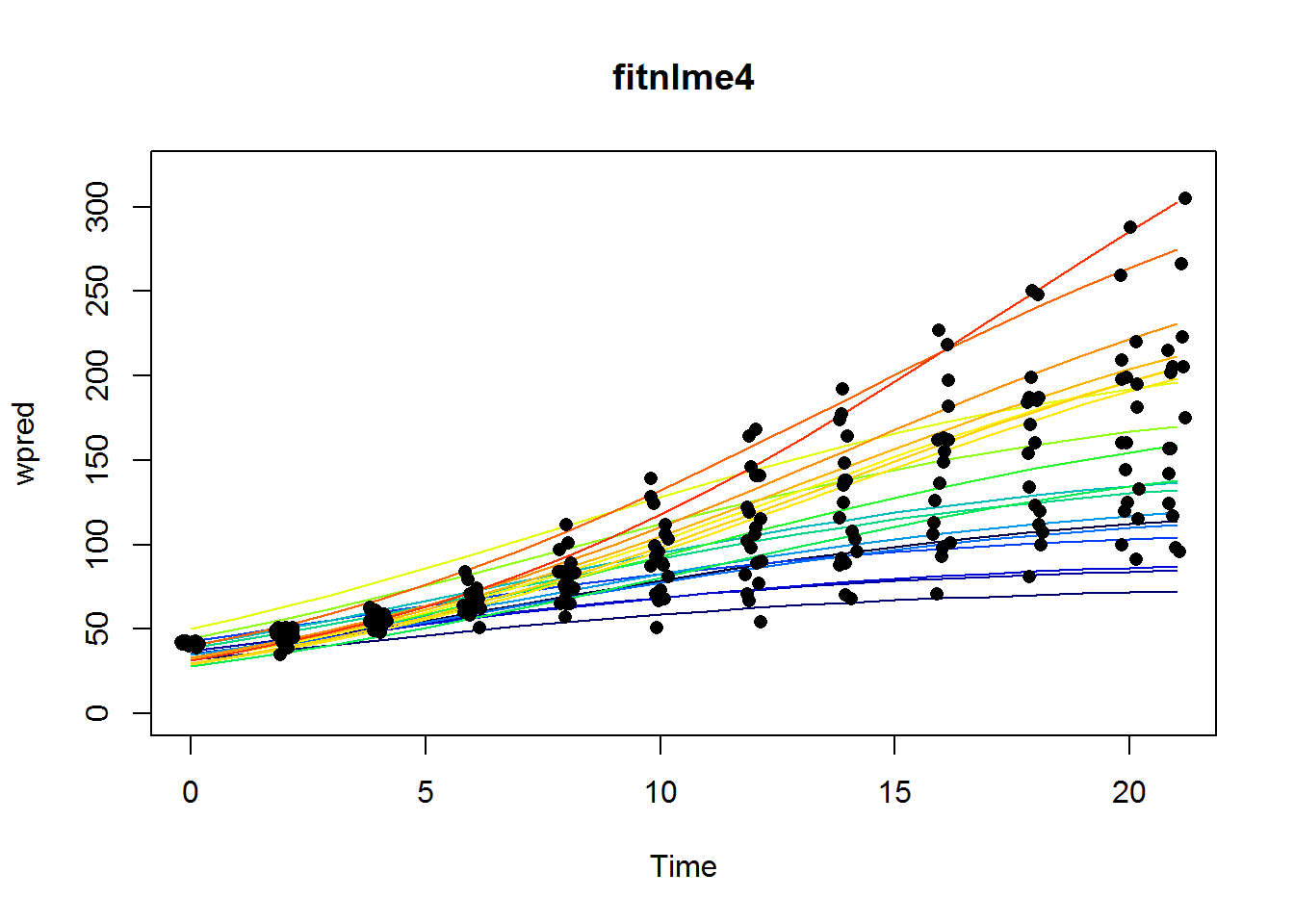### Other resources

• We have discussed non-linear regression before during R sessions, see: 2013-04-05 : Non-linear regression.

• A very good book on non-linear regression with R is Ritz and Streibig 2008 (online access on campus).

• It can be difficult to find the right non-linear model. I have yet to find a better alternative to a SAS-oriented guide to curve fitting, published in 1994 by the Province of British Columbia (download it from the Resources section on the HIE R site on sharepoint).

### Brief introduction

Basically, non-linear regression is used when you cannot use some sort of linear model. A model is linear when you can write it like this:

$Y = b_0 + b_1*X_1 + b_2*X_2 + b_3*X_3$

where Y is the dependent variable, and the X’s are the predictors, which can be any variable or some transformation thereof (for example, squared terms, two variables multiplied together, etc.). This model is linear in the coefficients b1, b2, etc, though it may contain terms that are non-linear in the X’s (such as squared terms of X).

An example of a non-linear model is the Chapman-Richards growth equation,

$Y = Y_{max}*(1-e^{-b*x})^c$

Here the coefficients (Ymax, b and c) occur non-linearly in the equation, and you cannot write this in a form similar to the linear model as shown above. We can look at the shape of this curve by using curve, like so:

# Define function
chapm <- function(x,Ymax,b,c=2.5)Ymax*(1-exp(-b*x))^c

# Plot (curve must use an x argument)
curve(chapm(x, Ymax=100, b=0.15, c=3), from=0, to=50, ylab="Y")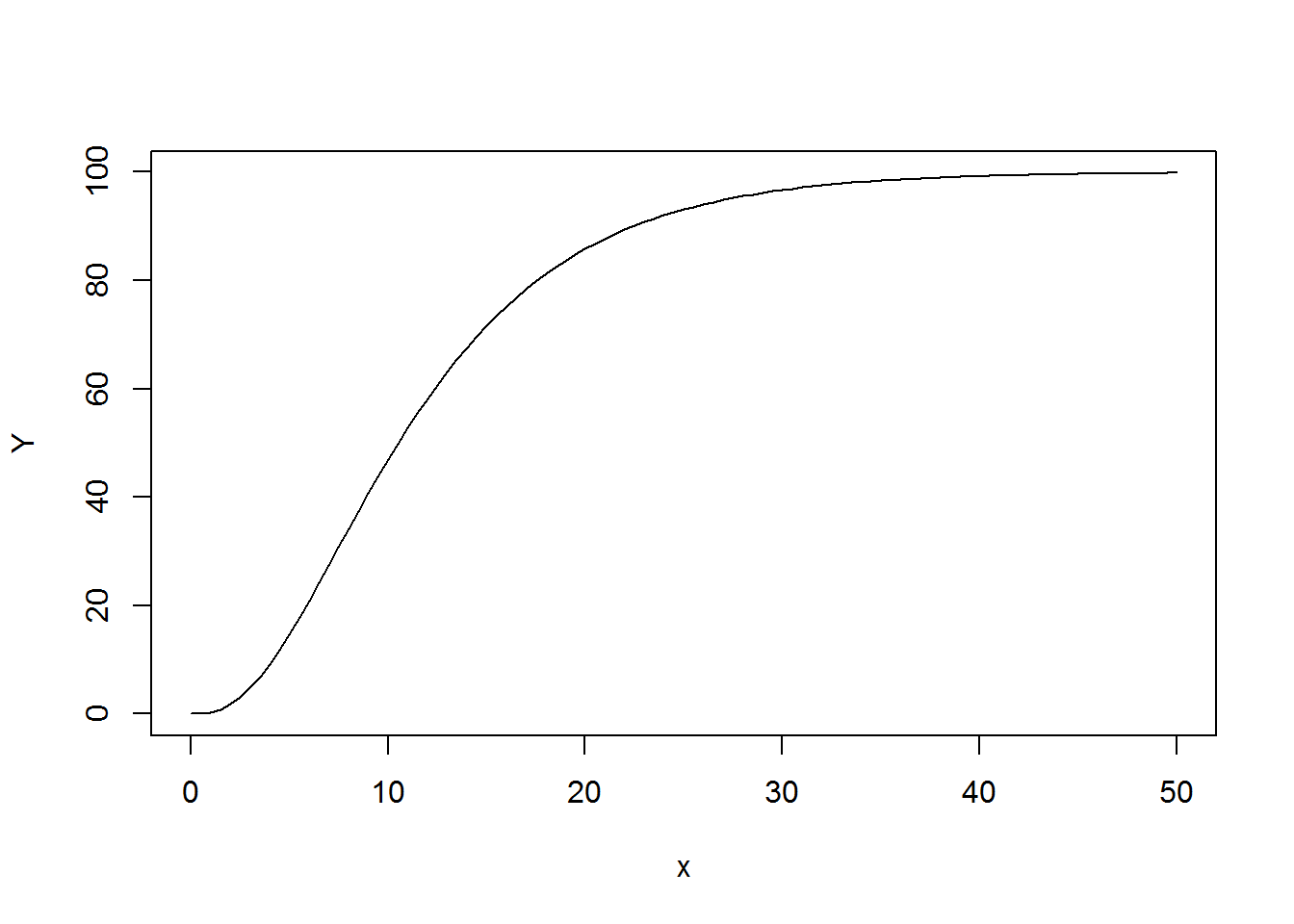We can use non-linear regression to fit curves like that one to data, which gives estimates of the coefficients Ymax, b and c. The majority of useful applications of non-linear regression are when you have an equation already specified (arising from theory), and you wish the estimate the parameters of the curve, or use the curve for prediction (as in lots of modelling applications).

In the following we use only the nls function, but point out nlsLM (package minpack.lm) for a very promising alternative, and nls2 (package of same name) for an alternative that can be used to find starting values.

### A simple non-linear model

The most annoying aspect of nls is that you have to specify starting values for the parameters, otherwise nls will in many cases not converge to a solution. Depending on the situation (and especially the equation to be fit, these starting values need to be quite good. Normally we plot the data, choose a curve to fit, and play with the coefficients until the fit is ‘close’.

Here we use the built-in Loblolly data (see ?Loblolly), which contains age and height of Loblolly pine (Pinus taeda), for several seedlots.

# We have chosen this function to fit.
fx <- function(x, Asym, R0, lrc)Asym+(R0-Asym)*exp(-exp(lrc)*x)

# Make plot and add a curve.
with(Loblolly, plot(age, height))
curve(fx(x, Asym=100, lrc=-3, R0=-8), add=T)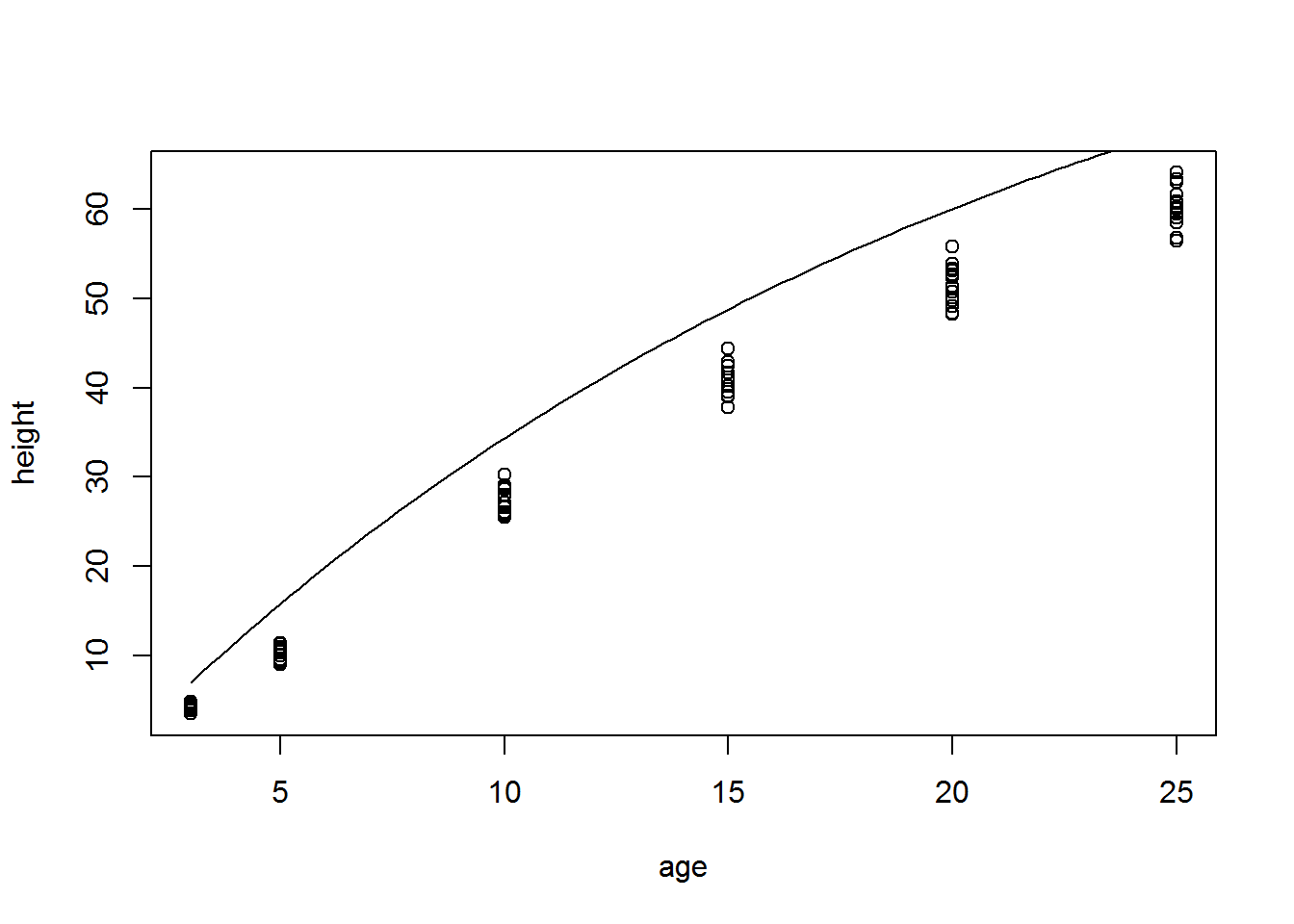That’s pretty close, so let’s see if nls can converge.

# Note the specification of the starting values.
nlsfit <- nls(height ~ fx(age, Asym, R0, lrc),
data=Loblolly,
start=list(Asym=100, lrc=-3, R0=-8))

Nothing was printed, which is good news. If it does not converge it will report an error.

Note that you do not have to write a function first and use it within nls, you can also write the equation in the formula interface of nls directly. However, it is usually convenient to have the function defined separately.

We can add the fitted curve to a plot in two ways. Here is the first, which uses the estimated coefficients and plugs them into the function we fit to the data.

# Save coefficients in a vector
p <- coef(nlsfit)

# Plot and add a curve. Note that 'p' is a named vector.
with(Loblolly, plot(age, height, xlim=c(0, 30), ylim=c(0,75)))
curve(fx(x, Asym=p["Asym"], R0=p["R0"], lrc=p["lrc"]),
add=T, col="red")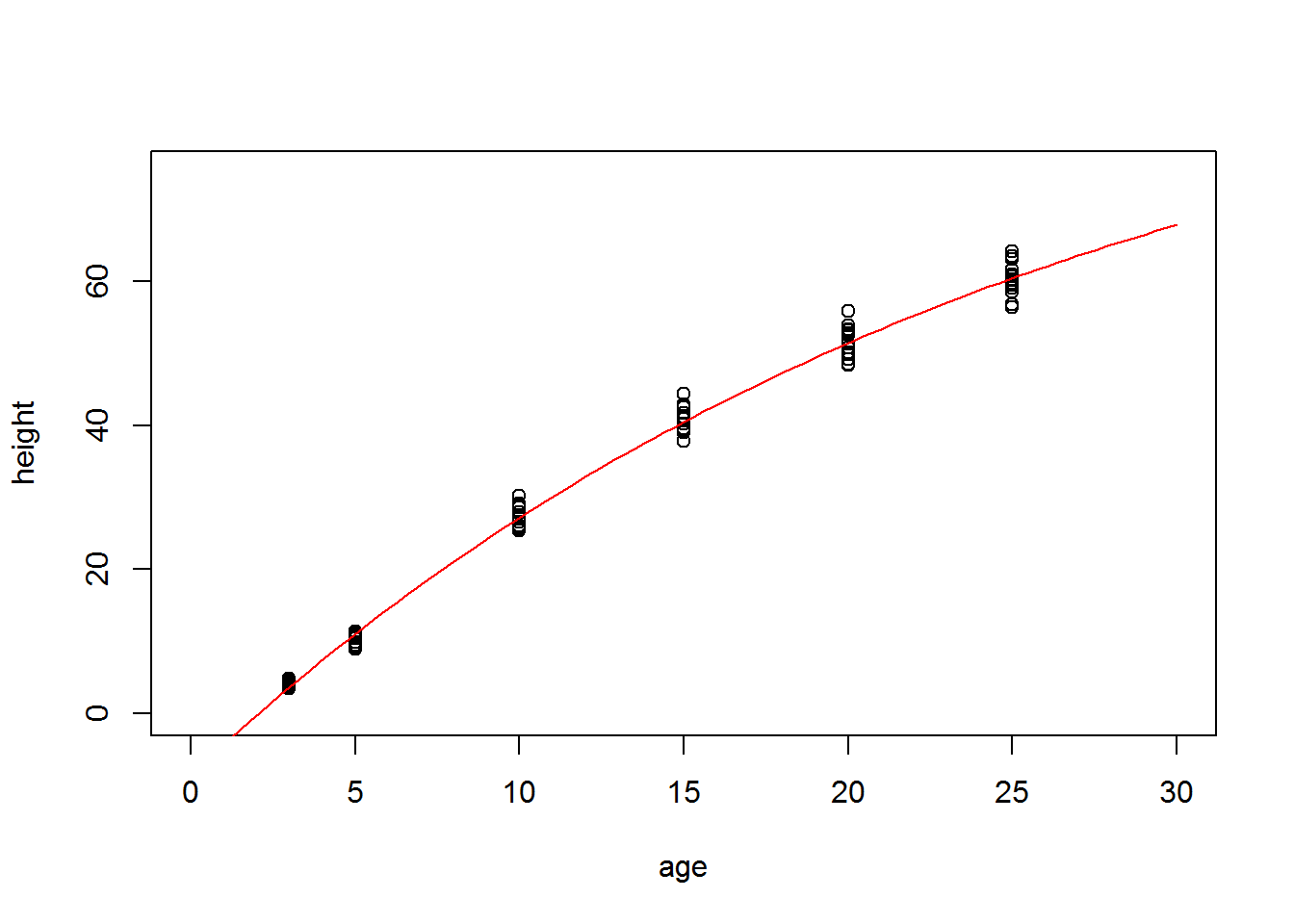The second method uses predict, which is sometimes easier to use (and is safer in the sense that it is more difficult to make mistakes in the code).

# Make dataframe with X variable that we wish to predict Y values for.
# Make sure it has the same name as in the dataframe we used to fit the model!
newdat <- data.frame(age=seq(2,25, length=101))

# Predict from the fitted model for the new dataframe.
newdat$heightpred <- predict(nlsfit, newdata=newdat) # Add a line. with(Loblolly, plot(age, height, xlim=c(0, 30), ylim=c(0,75))) with(newdat, lines(age, heightpred, col="blue"))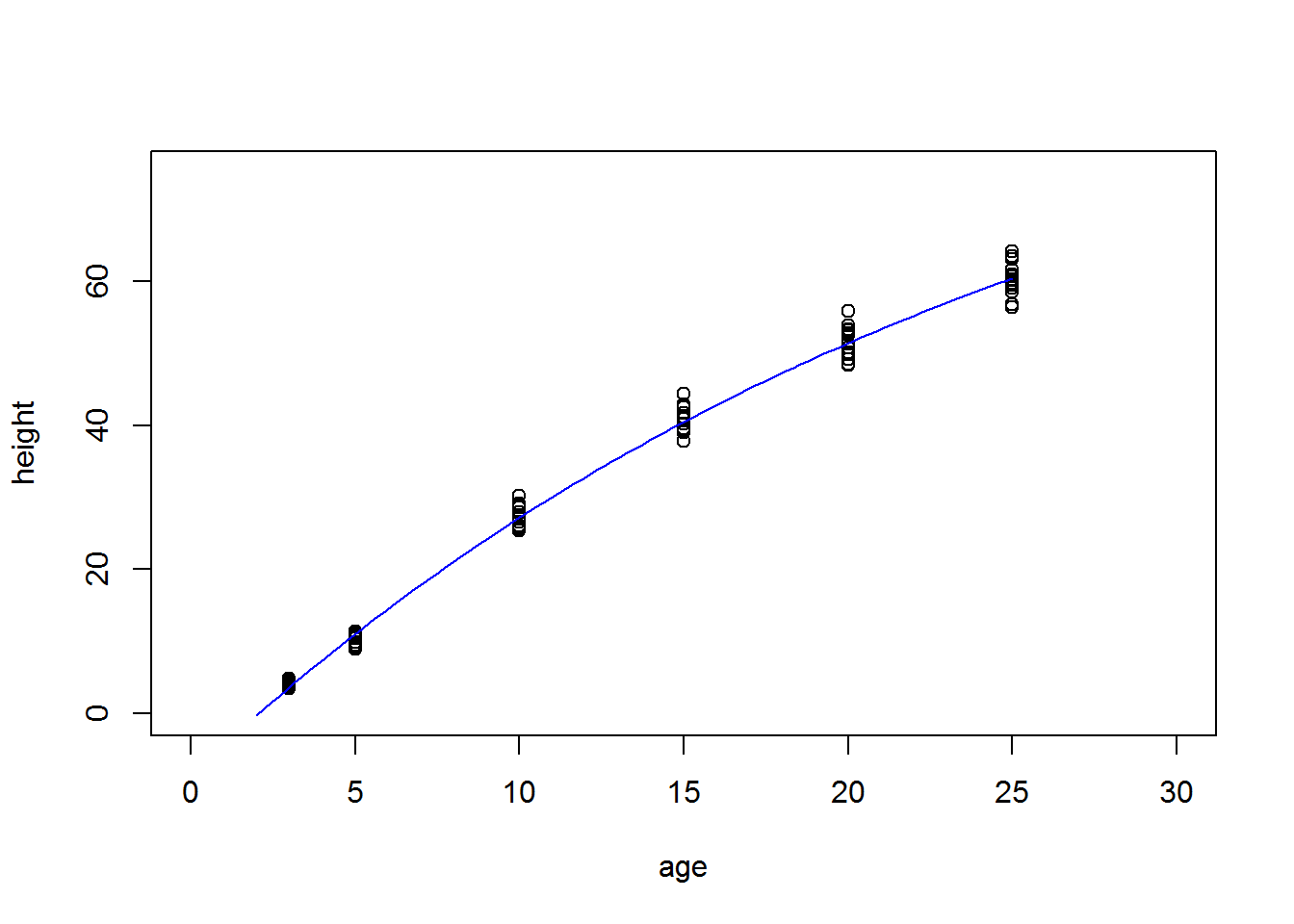Unlike the predict method for linear models (predict.lm in particular), we cannot estimate standard errors or confidence intervals for predictions (although predict.nls does have an argument se.fit which is actually ignored!). To do this, we rely once again on the bootstrap. Suppose we are interested in the predicted value of height at an age of 22.5 years, and do so by interpolating with the fitted nonlinear model. This example shows how to get a confidence interval for that estimate as well. library(car) # bootstrap resampling. The object b contains coefficients of the nonlinear model fitted to # 999 resampled datasets. b <- bootCase(nlsfit) # Note that we defined fx above, it is the nonlinear equation fit to the data. p225 <- fx(x=22.5, Asym=b[,"Asym"], lrc=b[,"lrc"], R0=b[,"R0"]) # The 2.5 and 97.5% quantiles give the confidence interval for the prediction. quantile(p225, probs=c(0.025, 0.975)) ## 2.5% 97.5% ## 55.39 56.86 ### Self-starting functions To avoid the annoying fiddling with starting values, we can use one of many ‘self-starting functions’. These are common non-linear models that do not require starting values. You can also develop these yourself, but this is tricky (see the reference to the book in ‘Other resources’ above for more information). I won’t go into much detail on these functions, but simply redo our example with the Loblolly data from above. I actually copied that function from the example under SSasymp, a self-starting asymptotic function. # Fit same model, using a self-starting model nlsfitSS <- nls(height ~ SSasymp(age, Asym, R0, lrc), data=Loblolly) The result should be exactly the same as above. It would be nice if someone were to make a compendium of all self-starting functions, including graphical illustrations of the functional shape and how it depends on the parameters. Summaries of the functions can be found in various places but a visual guide would be useful, and is lacking at the moment. ### Non-linear mixed effects models Next, we briefly look at adding random effects to a nonlinear model. Suppose we have repeated measured for a number of individuals, and would like to take into account the variation between individuals, rather than fitting one model for the entire dataset. In the following, I will show an example of a non-linear mixed effects model using the nlme package. I will use the built-in ChickWeight data, where a number of individual chicks were weighed daily for 21 days, while being fed on one of four diets. The question is, which diet causes the quickest growth of the chicks, and was the growth trajectory different by diet? First we visualize the data. Here I use the plotBy package, which I developed. Ssee this website for instructions to install it. library(plotBy) library(gplots) palette(rich.colors(20)) plotBy(weight ~ Time|Chick, type='l', data=subset(ChickWeight, Diet == "1"), legend=FALSE)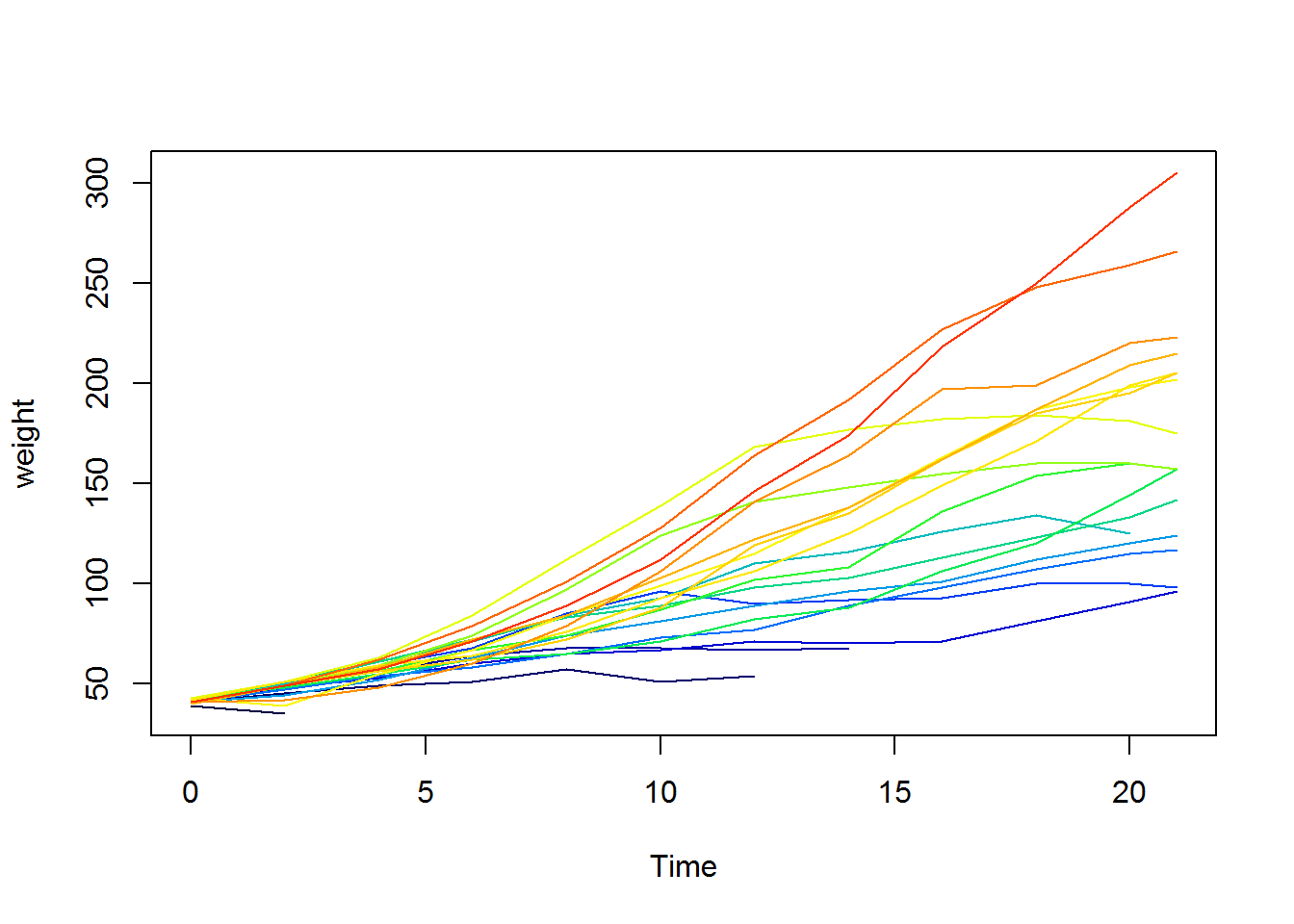Here I plotted the chicks for the first diet only. We see that the individual chicks: • differ a lot in their final weight • differ in the growth trajectory (the shape of the curve) Also, some chicks died after a few days. Clearly we cannot fit a single curve to this cloud of data, but we should be able to estimate a population average growth curve, and some measures of the variance between chicks. To do this, we can fit a mixed-effects model where the parameters of the model to be fit can vary between subjects (random effects), and between treatments. The latter would be a fixed effect because we are actually interested in their values, but the individual chick effect should be a random effect because we simply wish to account for this source of variation, but we are not really interested in how well chick nr 5 did compared to nr 6. The logistic growth model is quite useful here. First, let’s visualize to see how the parameters affect the shape of the curve. # We will use SSlogis shortly, but this is the curve itself: flogis <- function(x, Asym, xmid, scal)Asym/(1+exp((xmid-x)/scal)) # Four curves with different parameter values. curve(flogis(x, 200, 10, 2), from=0, to=21, lwd=2) curve(flogis(x, 100, 10, 2), from=0, to=21, lty=5, add=TRUE) curve(flogis(x, 200, 15, 2), from=0, to=21, col="red", add=TRUE) curve(flogis(x, 200, 10, 4), from=0, to=21, col="blue", add=TRUE)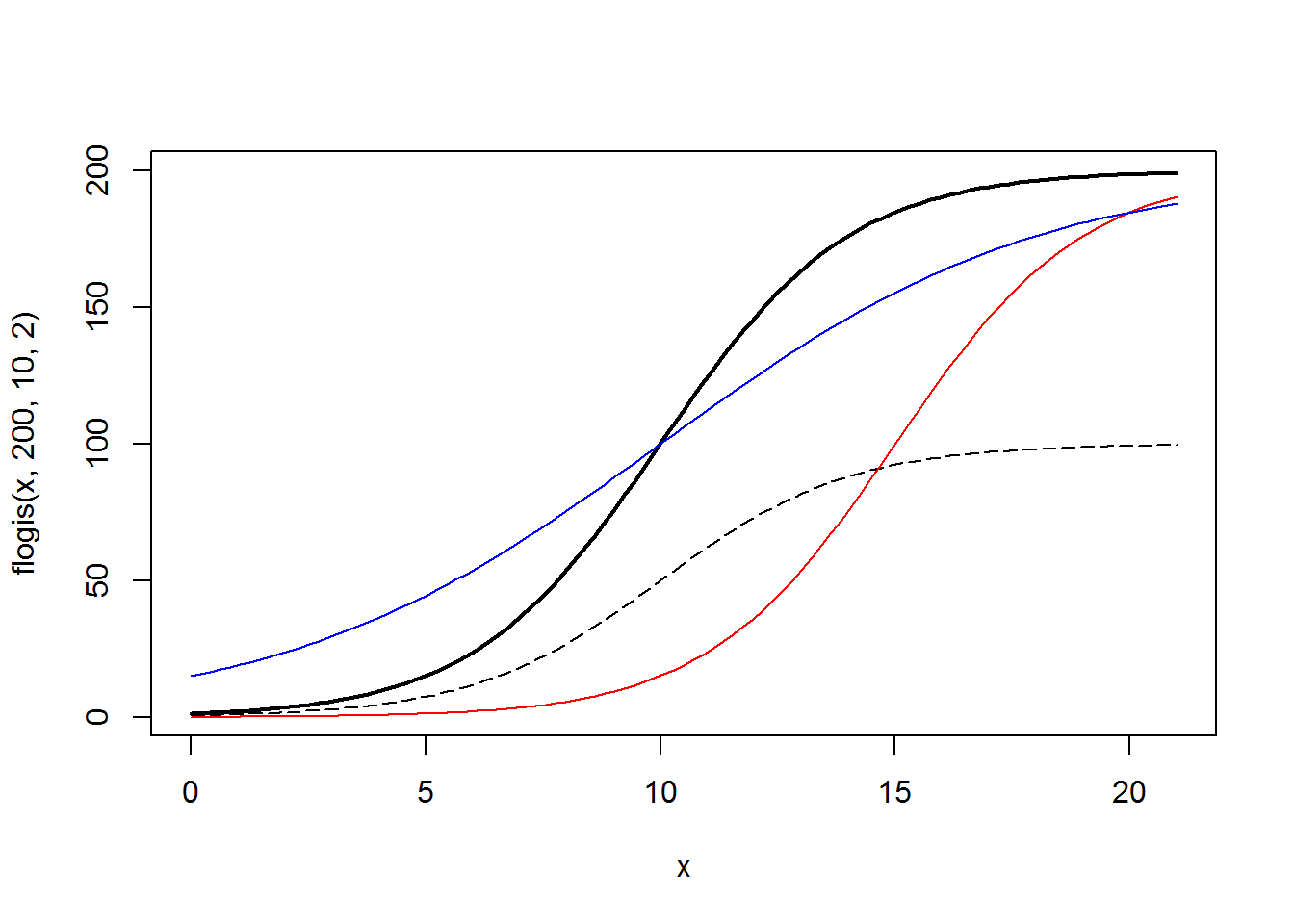Clearly, Asym affects the asymptote, xmid the inflection point, and scal is a shape parameter (note when I increased it - the blue curve above - the curve flattened). #### Fitting a model with one random effect Let’s start by fitting a non-linear mixed-effects model with only one random effect (for Asym), and one fixed effect (Asym is varied by Diet). The syntax for nlme is a bit strange: even though we will use a self-starting function (to avoid the common problem of not converging), we still have to specify the starting values. And because Asym will vary as a fixed effect by Diet, we have to specify four starting values, one for each Diet. To test whether Asym varies significantly by diet, it is appropriate to use a likelihood ratio test against a model that does not include the Diet effect. So, we first fit fitnlme0, which includes no Diet, and then fitnlme0, which includes diet as a fixed effect term for Asym. require(nlme) fitnlme0 <- nlme(weight ~ SSlogis(Time, Asym, xmid, scal), fixed=list(Asym + xmid + scal ~ 1), random = Asym ~ 1 | Chick, start=list(fixed=c(Asym=200,xmid=13,scal=4)), data=ChickWeight) fitnlme1 <- nlme(weight ~ SSlogis(Time, Asym, xmid, scal), fixed=list(Asym ~ Diet, xmid + scal ~ 1), random = Asym ~ 1 | Chick, start=list(fixed=c(Asym=c(150,200,250,300),xmid=13,scal=4)), data=ChickWeight) # Likelihood ratio test anova(fitnlme0, fitnlme1) ## Model df AIC BIC logLik Test L.Ratio p-value ## fitnlme0 1 5 4980 5001 -2485 ## fitnlme1 2 8 4968 5003 -2476 1 vs 2 17.45 6e-04 Clearly Diet is very significant (also note the AIC has decreased). Next, let’s look at what the fitted model looks like, and if it is successful at describing the most important patterns in the data. We use predict to get model predictions, and for nlme models, you have to specify whether to include the random effects or not. If you only want predictions for the fixed effects (sort of averaged over all individual chicks), specify level=0, otherwise leave it as is and the random effects will also be used. # Fixed effects predictions # Make dataframe with all combinations of Diet and Time. newdat <- expand.grid(Diet=levels(ChickWeight$Diet), Time=0:21)
newdat$wpred <- predict(fitnlme1,newdat,level=0) # Plot. I use jitter so not all data cover each other. palette(c("blue","red","forestgreen","darkorange")) with(ChickWeight, plot(jitter(Time), weight, pch=19, col=Diet, main="fitnlme1")) plotBy(wpred ~ Time|Diet, data=newdat, type='l', add=TRUE, lwd=2)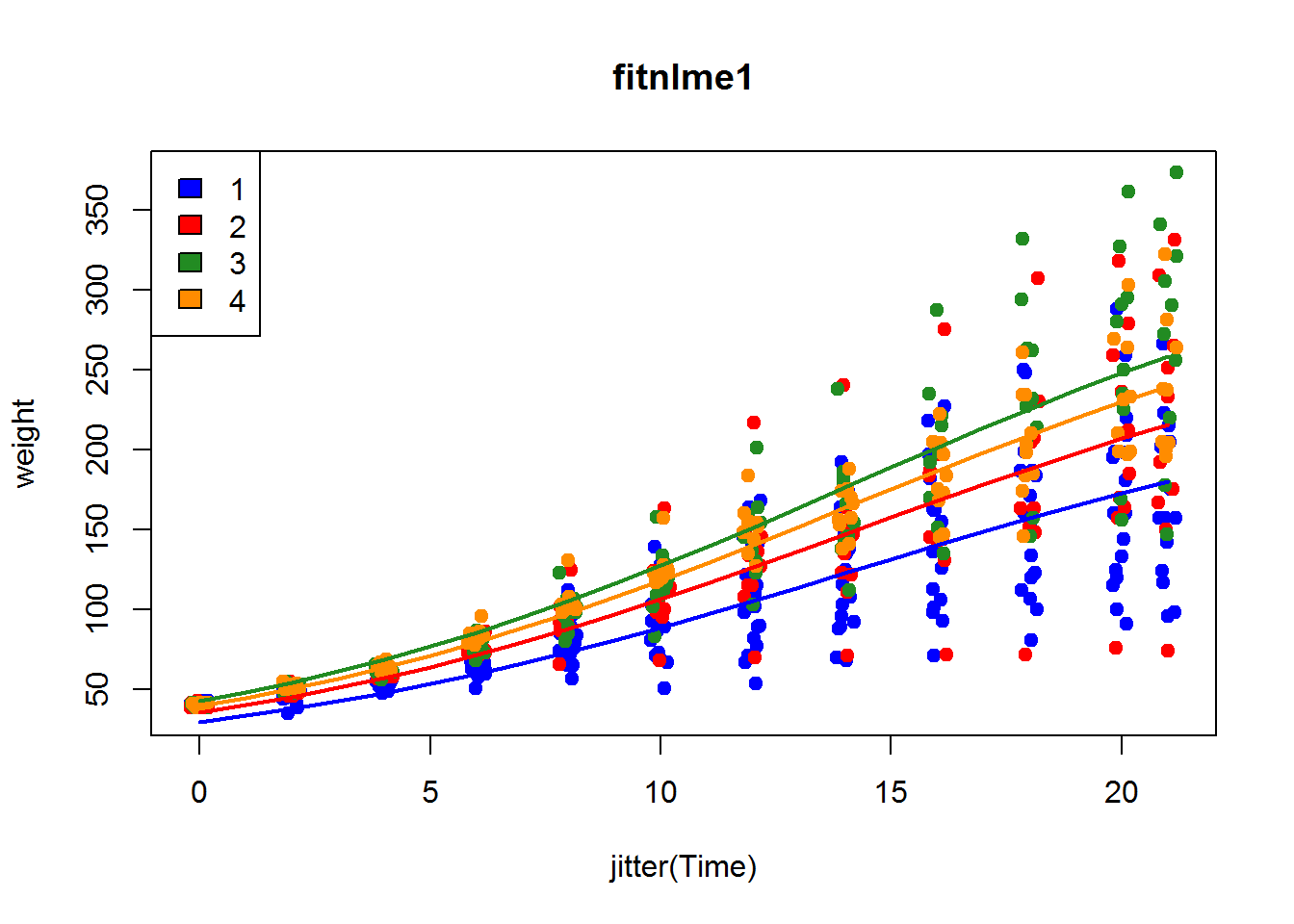Next we visualize the random effects. In this example I just use one diet, to keep things a bit simple. # Random effects, diet 1 chick1 <- droplevels(subset(ChickWeight, Diet == "1")) # Note that we now need the Chick number in here, otherwise predict won't work! newdat1 <- expand.grid(Diet="1", Chick=levels(chick1$Chick), Time=0:21)
newdat1$wpred <- predict(fitnlme1,newdat1) palette(rich.colors(nlevels(newdat1$Chick)))
plotBy(wpred ~ Time|Chick, data=newdat1, type='l',
ylim=c(0,320), legend=FALSE)
with(chick1, points(jitter(Time), weight, pch=16))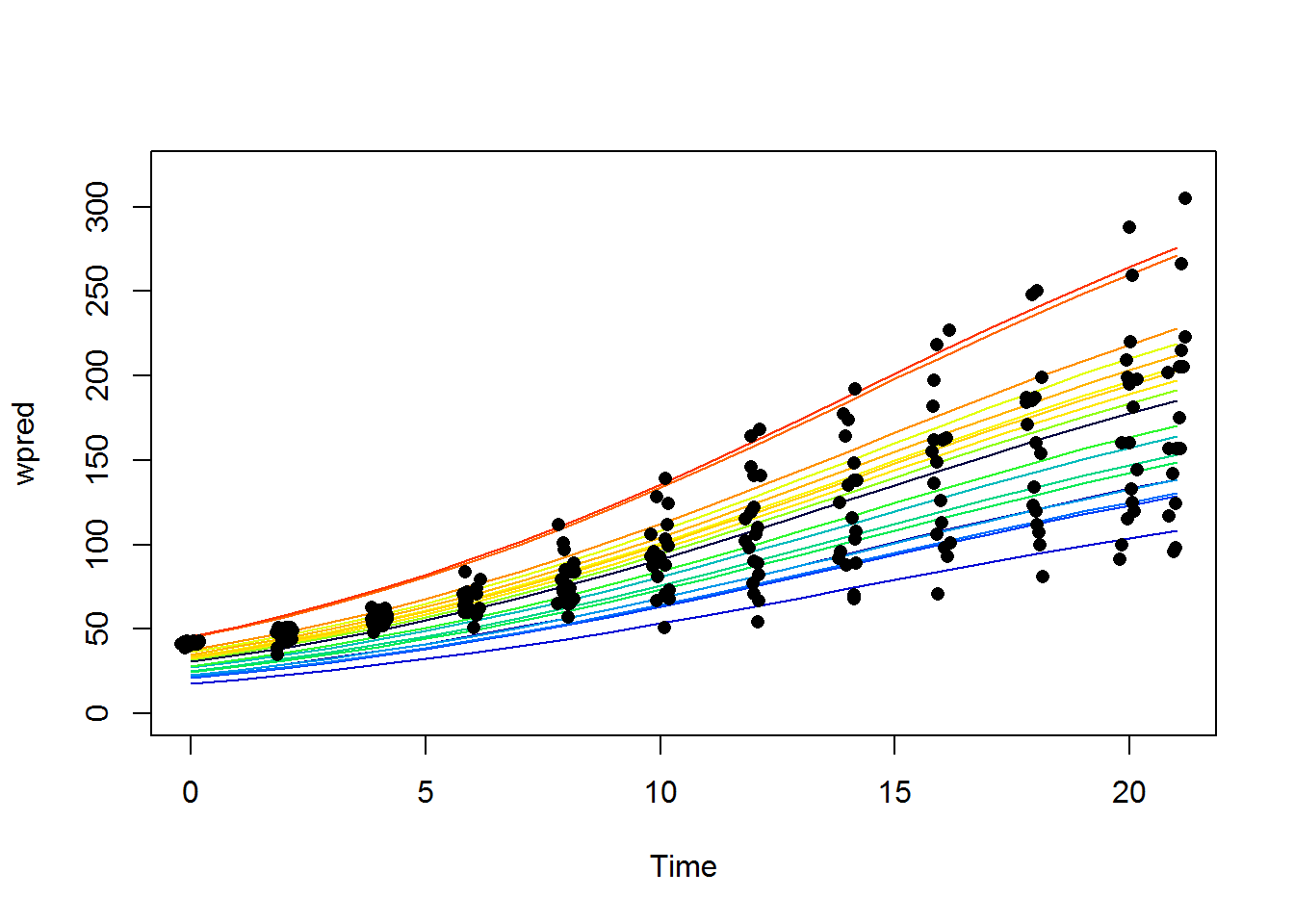From the graph we can see that some of the data structure is modelled OK, but the shapes of the curves are all wrong for the first 10 days or so. But we know the curves are more flexible, so if we also include random effects for the other parameters, it should gradually improve. If it does, the AIC should decrease (and the likelihood ratio test should be significant). In the following three examples, I increase the model complexity one step at a time, and see what happens. See for yourself how and if the model improves.

### Increasing the model complexity

This model includes random effects for Asym and xmid.

fitnlme2 <- nlme(weight ~ SSlogis(Time, Asym, xmid, scal),
fixed=list(Asym ~ Diet, xmid + scal ~ 1),
random = Asym +xmid ~ 1 | Chick,
start=list(fixed=c(Asym=c(150,200,250,300),xmid=13,scal=4)),
data=ChickWeight)
anova(fitnlme1, fitnlme2)
##          Model df  AIC  BIC logLik   Test L.Ratio p-value
## fitnlme1     1  8 4968 5003  -2476
## fitnlme2     2 10 4448 4492  -2214 1 vs 2   524.2  <.0001
newdat$wpred2 <- predict(fitnlme2,newdat,level=0) palette(c("blue","red","forestgreen","darkorange")) with(ChickWeight, plot(jitter(Time), weight, pch=19, col=Diet,main="fitnlme2")) plotBy(wpred2 ~ Time|Diet, data=newdat, type='l', add=TRUE, lwd=2)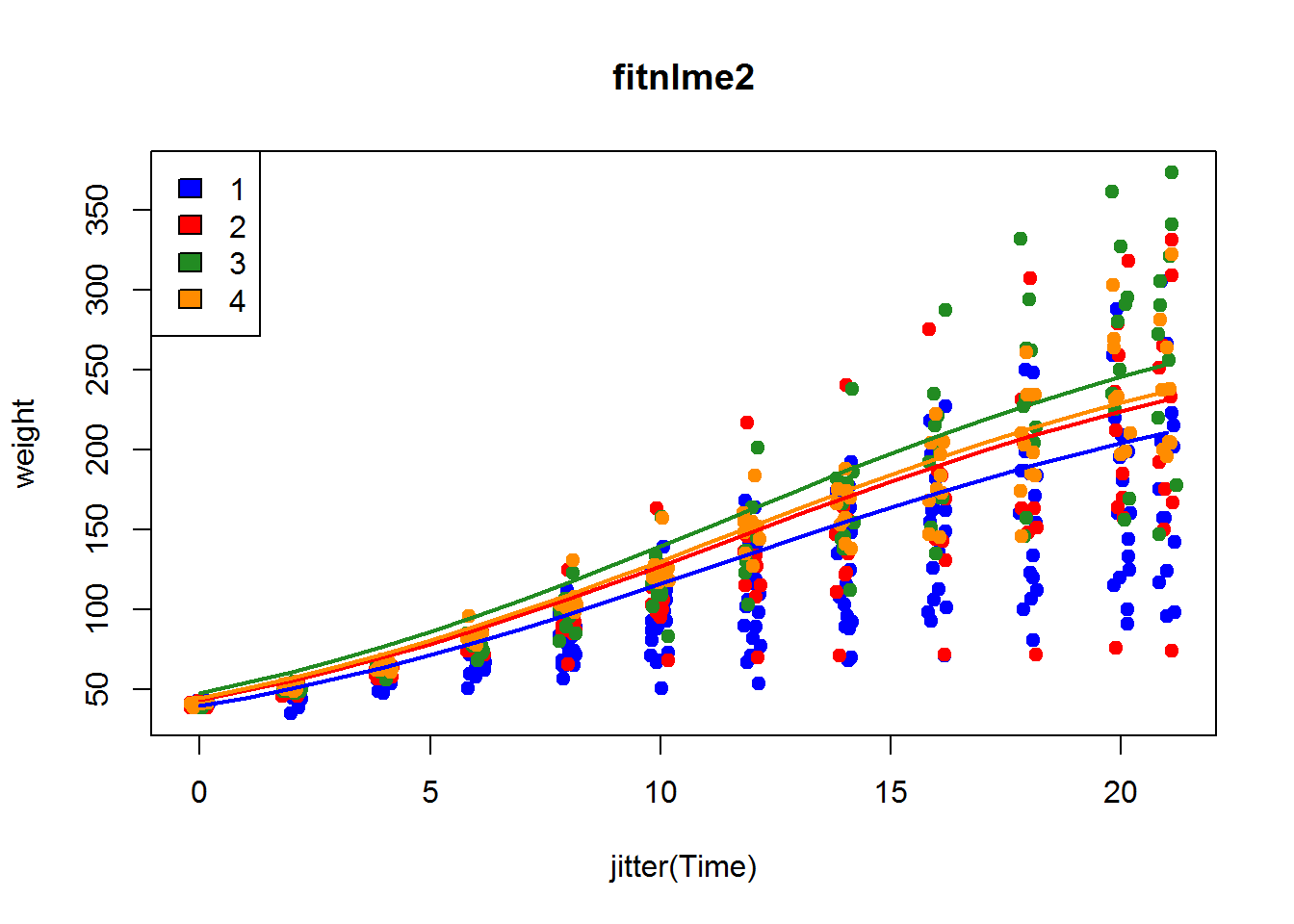# Random effects, diet 1 newdat1$wpred <- predict(fitnlme2,newdat1)

palette(rich.colors(nlevels(newdat1$Chick))) plotBy(wpred ~ Time|Chick, data=newdat1, type='l', main="fitnlme2", ylim=c(0,320), legend=FALSE) with(chick1, points(jitter(Time), weight, pch=16))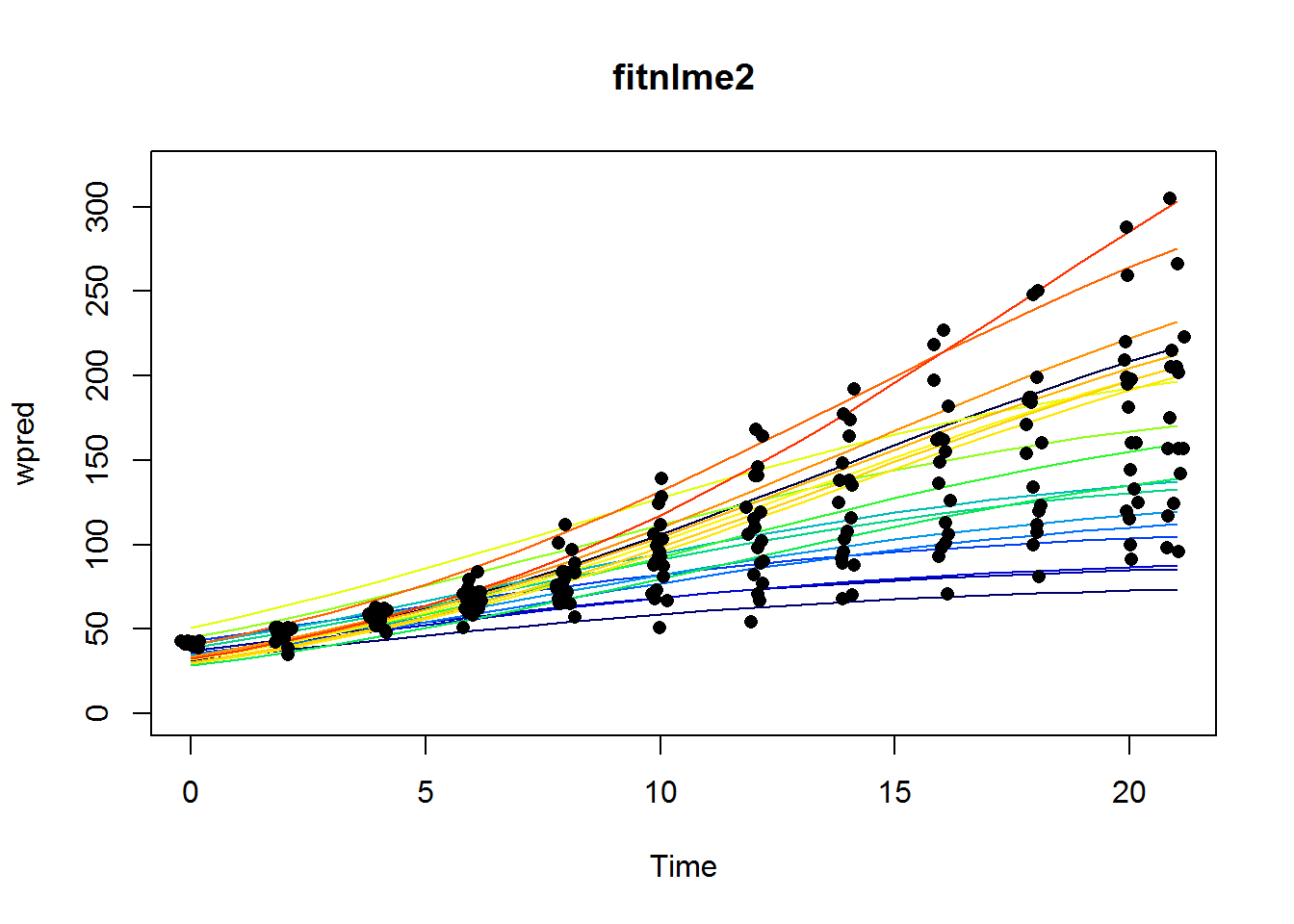This model includes random and fixed effects for both xmid and Asym. fitnlme3 <- nlme(weight ~ SSlogis(Time, Asym, xmid, scal), fixed=list(Asym + xmid ~ Diet, scal ~ 1), random = Asym +xmid ~ 1 | Chick, start=list(fixed=c(Asym=c(150,200,250,300),xmid=c(10,10,10,10),scal=4)), data=ChickWeight) anova(fitnlme2, fitnlme3) ## Model df AIC BIC logLik Test L.Ratio p-value ## fitnlme2 1 10 4448 4492 -2214 ## fitnlme3 2 13 4443 4500 -2209 1 vs 2 10.53 0.0145 # Fixed effects newdat$wpred3 <- predict(fitnlme3,newdat,level=0)
palette(c("blue","red","forestgreen","darkorange"))

with(ChickWeight, plot(jitter(Time), weight, pch=19, col=Diet, main="fitnlme3"))
plotBy(wpred3 ~ Time|Diet, data=newdat, type='l', add=TRUE, lwd=2)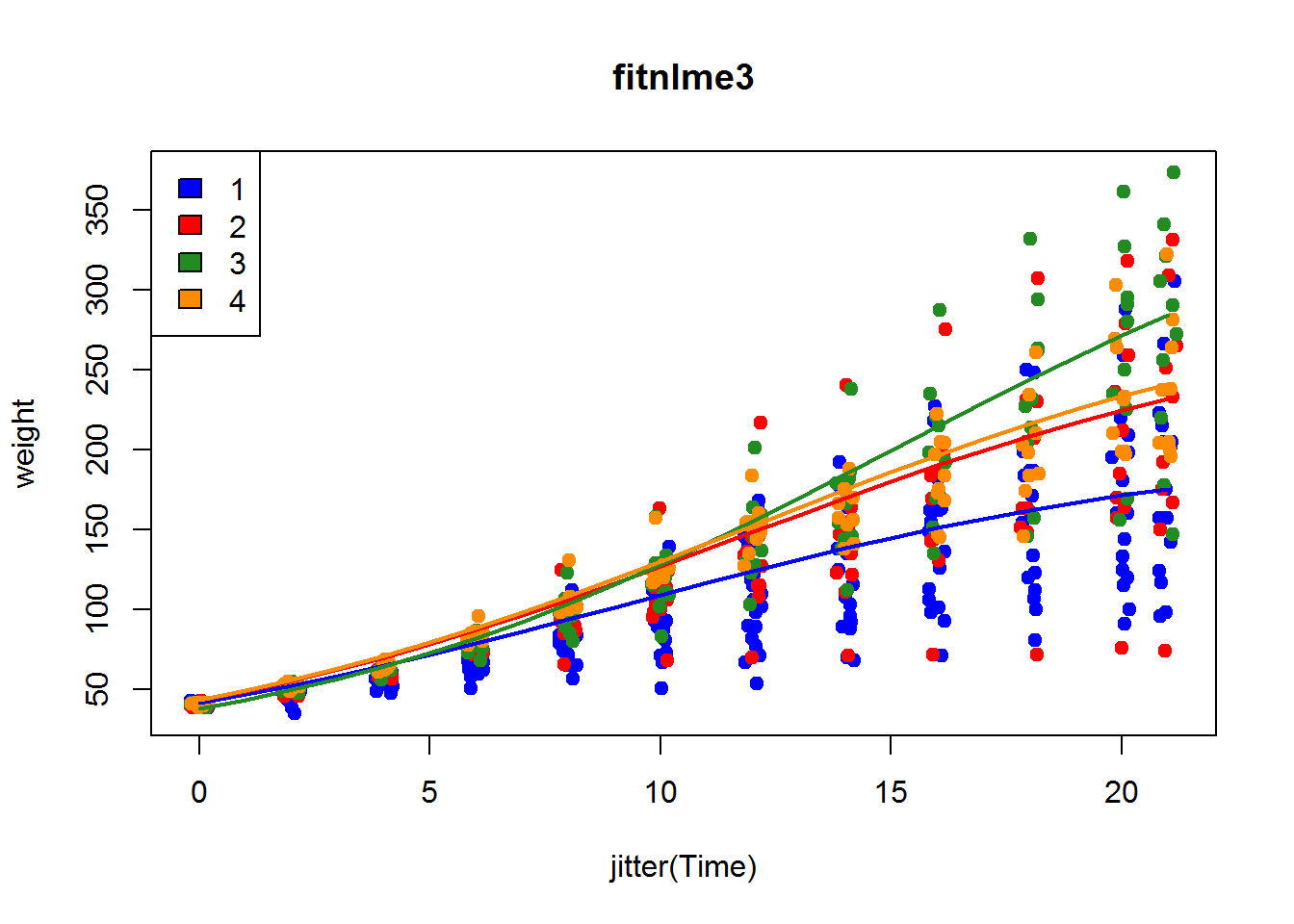# Random effects
newdat1$wpred <- predict(fitnlme3,newdat1) palette(rich.colors(nlevels(newdat1$Chick)))
plotBy(wpred ~ Time|Chick, data=newdat1, type='l', main="fitnlme3",
ylim=c(0,320), legend=FALSE)
with(chick1, points(jitter(Time), weight, pch=16))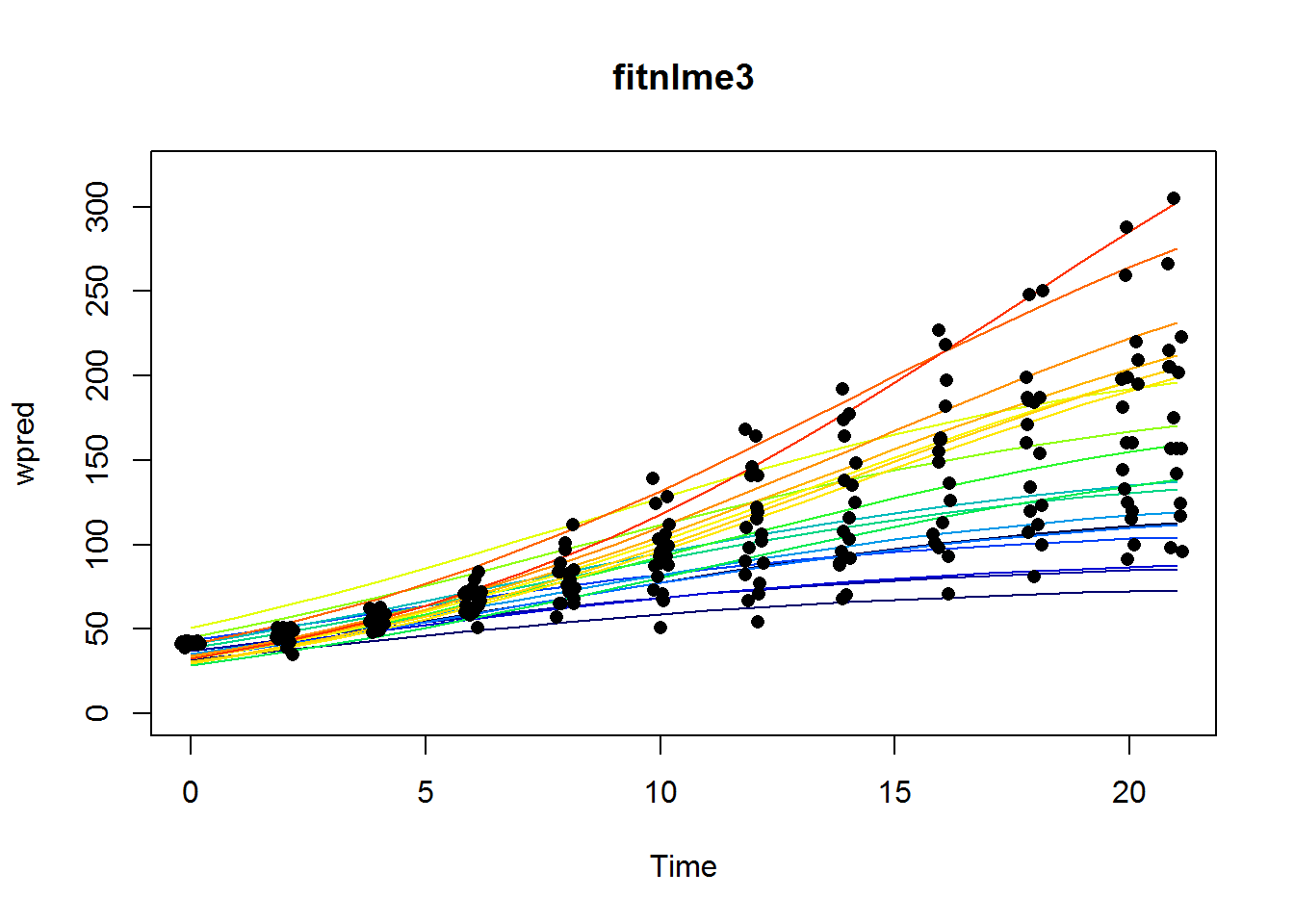And finally, this model includes all random (but not scal, otherwise the model converges very slowly) and fixed effects.

fitnlme4 <- nlme(weight ~ SSlogis(Time, Asym, xmid, scal),
fixed=list(Asym + xmid + scal ~ Diet),
random = Asym + xmid ~ 1 | Chick,
start=list(fixed=c(Asym=c(150,200,250,300),xmid=c(10,10,10,10),scal=c(4,4,4,4))),
data=ChickWeight)
anova(fitnlme3, fitnlme4)
##          Model df  AIC  BIC logLik   Test L.Ratio p-value
## fitnlme3     1 13 4443 4500  -2209
## fitnlme4     2 16 4446 4516  -2207 1 vs 2   3.045  0.3847
# Fixed effects
newdat$wpred4 <- predict(fitnlme4,newdat,level=0) palette(c("blue","red","forestgreen","darkorange")) with(ChickWeight, plot(jitter(Time), weight, pch=19, col=Diet, main="fitnlme4")) plotBy(wpred4 ~ Time|Diet, data=newdat, type='l', add=TRUE, lwd=2)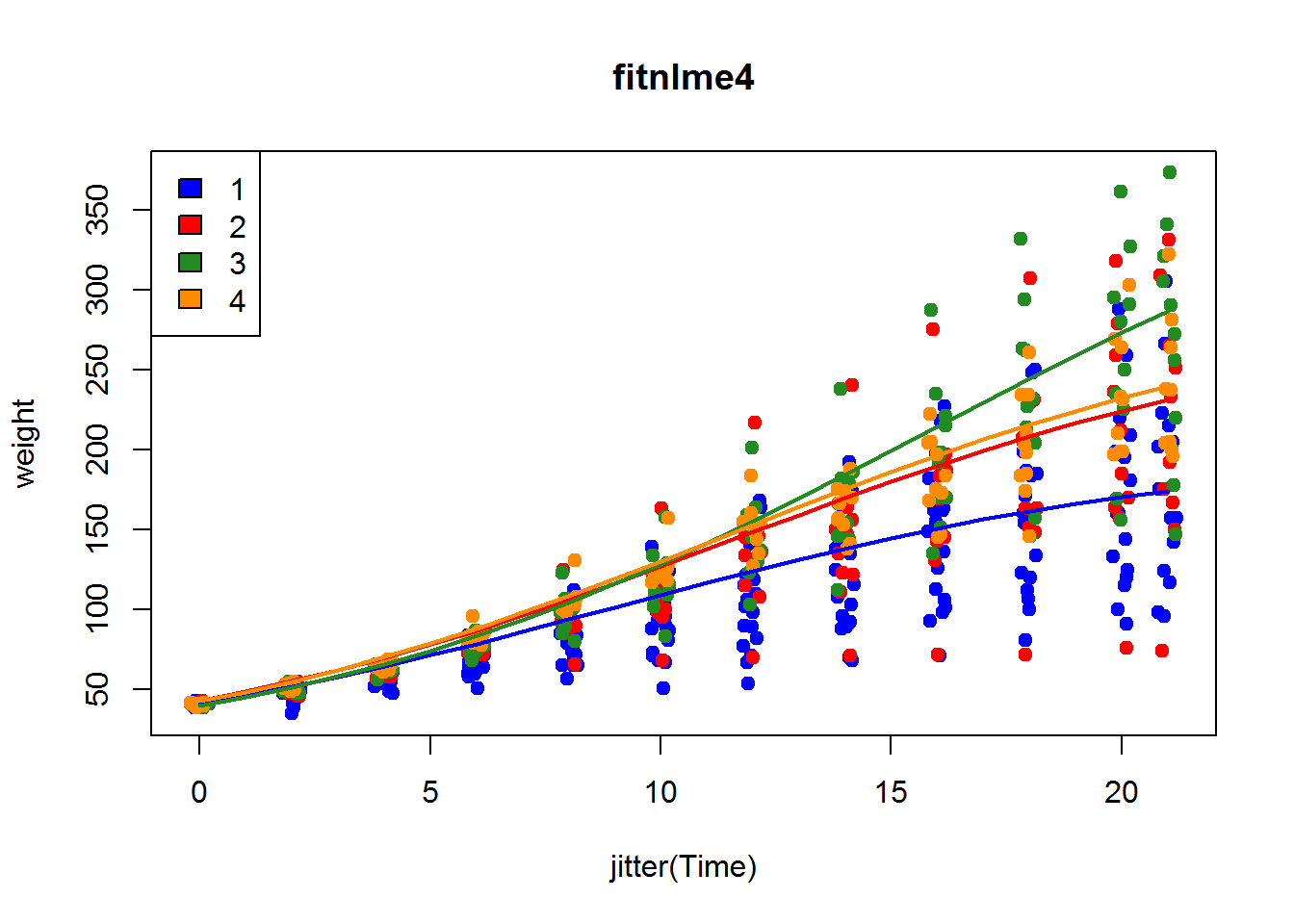# Random effects newdat1$wpred <- predict(fitnlme4,newdat1)

palette(rich.colors(nlevels(newdat1\$Chick)))
plotBy(wpred ~ Time|Chick, data=newdat1, type='l', main="fitnlme4",
ylim=c(0,320), legend=FALSE)
with(chick1, points(jitter(Time), weight, pch=16))